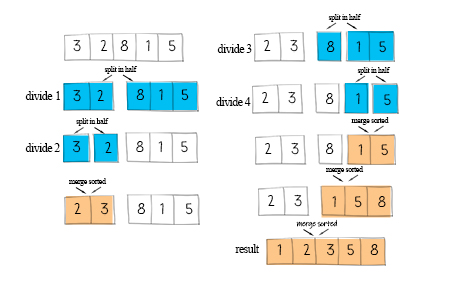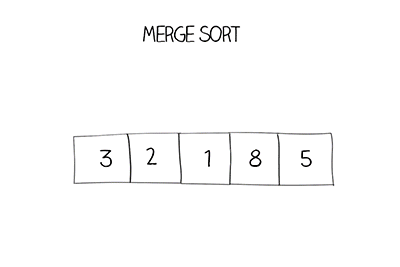Merge sort divides the array in half recursively until to single item. Then merges two sorted arrays until all elements are sorted. Merge sort is similar to quicksort in the way they both use divide and conquer technique. The difference is that merge sort always divides the array from the middle. Another facts is that it merges two sorted arrays by using merge method.The merge method is to compare each element in two sorted array one by one, put the smaller one in the third array until one of arrays reaches the end. Then copy the rest of unfinished array to the third array. This requires an additional array in memory, equal in size to the one being sorted.

Merge sort is an efficient sorting technique. It has O(nlogn) complexity. The downside is that it requires extra space for the additional array.

## Doodle